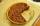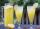# Pizza 5

You have 2/4 of a pizza and you want to share it equally between 2 people how much pizza does each person get?

Result

x =  0.25

#### Solution:Leave us a comment of example and its solution (i.e. if it is still somewhat unclear...):

Showing 0 comments:Be the first to comment!#### To solve this example are needed these knowledge from mathematics:

Need help calculate sum, simplify or multiply fractions? Try our fraction calculator.

## Next similar examples:

1. ChocolateChildren break chocolate first to third and then every part of another half. What kind got each child? Draw a picture. What part would have received if each piece have halved?
2. WithdrawalIf I withdrew 2/5 of my total savings and spent 7/10 of that amount. What fraction do I have in left in my savings?
3. Cake 71/3 of a cake shared with 4 people. What share of the whole cake has each people?
4. PieVili ate three pieces of pie . If it piece is 1/8 how much pie did he eat?
5. In fractionsAn ant climbs 2/5 of the pole on the first hour and climbs 1/4 of the pole on the next hour. What part of the pole does the ant climb in two hours?
6. Water 31Richard takes 3 1/6 liters of water before noon and 2 3/5 liters of water after noon. How many litres of water does Richard consume a day ?
7. Fractions 4How many 2/3s are in 6?
8. Lengths of the poolMiguel swam 6 lengths of the pool. Mat swam 3 times as far as Miguel. Lionel swam 1/3 as far as Miguel. How many lengths did mat swim?
9. Fraction and a decimalWrite as a fraction and a decimal. One and two plus three and five hundredths
10. Mixed2improperWrite the mixed number as an improper fraction. 166 2/3
11. Product of two fractionsProduct of two fractions is 9 3/5 . If one of the fraction is 9 3/7. Find the other fraction.
12. Fraction to decimalWrite the fraction 3/22 as a decimal.
13. ZdeněkZdeněk picked up 15 l of water from a 100-liter full-water barrel. Write a fraction of what part of Zdeněk's water he picked.
14. LemonadeHow many 1/4 cup servings are in 2 and 1/3 cups of lemonade?
15. Unit rateFind unit rate: 6,840 customers in 45 days
16. Math classificationIn 3A class are 27 students. One-third got a B in math and the rest got A. How many students received a B in math?
17. HusseinHussein owns 450000. He spent at the bookstore 2 over 9 to buy some books and tales. He paid 3 over 5 of his money to buy his math book. Then he paid his money to buy stories diagram ; a. Calculate the remaining amount of money with Hussein? b. Hussein l